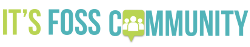# Shell - scripting - signed integer to unsigned

OK - I wrote a shell script this morning to run as a daily countdown… but the integer result I get is a negative value - i.e. a “signed integer”… I want an unsigned integer…

When I try to reformat it using `printf "%u"` I get some weird number.

Background, U.S. band Tool are releasing their first new album in 13 years on 30/08/2019 - and I wanted to calculate the number of days between now - and then run a “countdown” of days: I’m a bit “okay” with the signed output - but I’d rather just a number, unsigned.

``````#!/usr/bin/env bash
# Calculate numdays till new Tool album :
FONT=big
END="190830"
while true
do
clear
export TD=\$(date '+%y%m%d')
# echo \$TD
# echo \$(( (\$(date --date=\$TD +%s) - \$(date --date="190830" +%s) )/(60*60*24) ))
TENKDAYS=`echo \$(( (\$(date --date=\$TD +%s) - \$(date --date="\$END" +%s) )/(60*60*24) ))`
# TENKDAYS=\$((\$TENKDAYS + \$TENKDAYS))
UNSIGN=\$(printf "%u" \$TENKDAYS)
echo " ------------------------------ "
echo ""
echo \$TENKDAYS days till new tool album....
echo ""
echo "   \$TENKDAYS !!!" | figlet -f \$FONT
echo ""
echo " ------------------------------"
sleep 60
done
``````

e.g. today it returns “-51”… I’d rather just see “51”…

If I use \$UNSIGN result from " - I get some huge long number (e.g. 18446744073709551566)…

• update -
Note - I kinda resolved this by converting the signed int to a string - then used awk to remove the “-” if the value is less than zero… but I’d rather figure out how to convert the signed int to unsigned…

• update 2 -
Just typing this post I realised another possible solution - subtract -51 from 0 :
`echo \$((0 - -51))`

2 Likes

``````export TD=\$(date '+%y%m%d')
echo \$(( (\$(date --date=\$TD +%s)
``````

I believe that it can be replaced with date +%s

Also note The %s returns utc seconds since 1 Jan 1970 Do you want to round it to a midnight time?
but ```
date --date="\$END" +%s is larger than date +%s # that it is larger than your \$TD because it is today at midnight.
perhaps you want “date --date=”\$END" +%s" as being today at midnight being the left argument.

2 Likes

Also if number is negative multiply by -1. (-51 * -1) = 51.
From the old Algebra class; negative times negative equals positive.

1 Like

Thanks first responders- yeah I got it arse about…

Should be \$END less \$TDI was always crap at maths at school… even worse at Uni, failed comp-sci maths unit first attempt, failed exam 2nd attempt (but was the only unit you didn’t need to pass the exam to get a pass mark for semester)…

1 Like